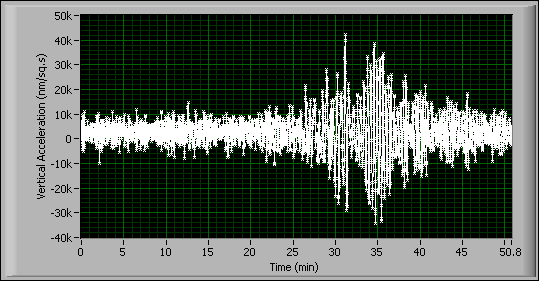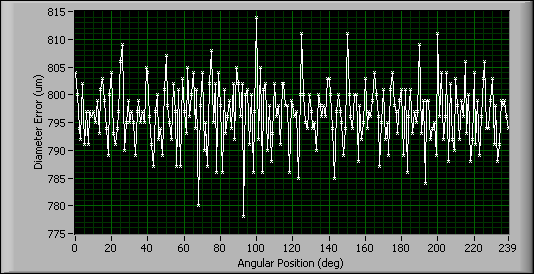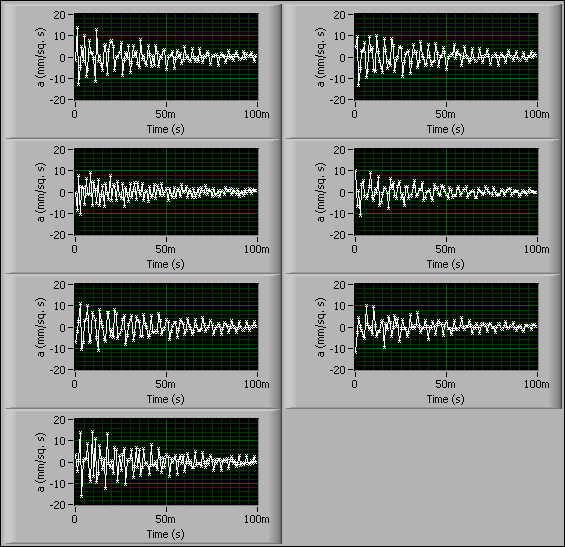# Introduction to Time Series Analysis (Advanced Signal Processing Toolkit)

LabVIEW 2014 Advanced Signal Processing Toolkit Help

Edition Date: June 2014

Part Number: 372656C-01

»View Product InfoDownload Help (Windows Only)

A time series is a sequence of observed values ordered through time. For example, the air temperature in meteorological science, blood pressure in biomedical science, or vibration in mechanical engineering or civil engineering are examples of a time series. Time series analysis uses a collection of systematic approaches to extract information about the characteristics of a physical system that generates time series. Approaches to time series analysis include estimating statistical parameters, building dynamic models, performing correlations, computing the power spectral density (PSD), and others.

Generally, a time series contains the following information:

• The characteristics of the time series, such as amplitude, spectral content and other statistical characteristics.
• The native characteristics or structural parameters of a system that generates the time series, for example, the natural frequency and damping of a civil structure.
• The characteristics of the input or stimulus to the physical system that generates the time series.

## Continuous Time Series and Discrete Time Series

In nature, physical quantities such as temperature, pressure, and light intensity change continuously. Observations of these values form a continuous time series.

Given a continuous time series, you can digitize the values at a specified time interval to obtain a discrete time series. The following figure shows the seismograph of the Kobe earthquake, recorded at Tasmania University, Hobart, Australia on January 16, 1995. In this figure, the continuous earthquake vibration signal is sampled at a one-second interval to form a discrete time series.Note  The data source in the previous figure is from the Time Series Data Library.

## Time-Ordered Series and Spatial-Ordered Series

Time series can be ordered not only through time but also through other physical units. For example, you can obtain a discrete time series ordered versus angular position by sampling the diameter of a spindle as a function of angle.

The following figure shows an example of the diameter error as a function of angle of a spindle during a lathe machining process. The diameter error generates a discrete time series ordered versus angular position.## Univariate Time Series and Multivariate Time Series

You can collect observed values from a single source or simultaneously from two or more sources. Single-source observations generate univariate time series, and multi-source observations form multivariate time series, or vector time series. For example, you can obtain a multivariate time series by recording the values of pressure, flow, and temperature simultaneously in an industrial process.

The following figure shows an example of the vibration signals from a steel-reinforced concrete beam. The signals are acquired simultaneously from seven acceleration sensors located at different positions on the beam.## Stationary Time Series and Nonstationary Time Series

In theory, given a behavioral model for a system, you can predict future values of a time series measured from that system, based on past observations. However, in practice, physical systems are affected by many kinds of disturbances, so the predicted values always reflect the stochastic, or statistical, characteristic of a time series.

Generally speaking, if the statistical characteristic of a time series contains no systematic change, the time series is stationary. Otherwise the time series is nonstationary.

## Time Series Analysis Objectives

Time series analysis is useful when you want to extract information from a time series, to discover the characteristics of a physical system that generates the time series, to predict the changes of a time series, or to improve control over the physical system. The objectives of time series analysis are as follows:

• Description—You can use the time series analysis methods to obtain descriptive or statistical measures of a time series. For example, to measure the trends or periodicity, you can plot the time series. To measure the symmetry of a time-series amplitude distribution, you can examine a histogram of the amplitude of a time series.
• Explanation—You can use the observed variation of a time series to explain the variation of a related time series, which can help you understand the nature of the relationship between the two signals. For example, you can explain the dynamic properties of a physical system by analyzing the input time series to and output time series from the system.
• Prediction—You can use observed values to predict the future values of a time series. For example, you can predict the future variation of a time series in an industrial process and make sure the process is working properly.
• Control—You can use the predicted values of a time series to determine appropriate corrective actions that you take to specify optimal settings for the controller and keep a physical system or process operating properly.

Time series exist in many application areas, ranging from economics to engineering. The LabVIEW Time Series Analysis Tools focus more on the applications in engineering. Use the Time Series Analysis VIs to analyze or process a time series.

Not Helpful